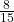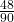Question

Represent 0.533 (only the last 3 is bar number) into a rational number

1.Step-by-step explanation:

We require 2 equations with the repeating 3 placed after the decimal point.

let x = 0.5333.. ( multiply both sides by 10 and 100 )

10x = 5.333… → (1)

100x = 53.333… → (2)

Subtract (1) from (2) tus eliminating the repeating 3

90x = 48 ( divide both sides by 90 )

x ==